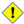# 8.1. 操作符

=

 ``` 1 var=27 2 category=minerals # 在"="之后是不允许出现空白字符的.`````` 1 # = 在这里是测试操作符 2  3 if [ "\$string1" = "\$string2" ] 4 # if [ "X\$string1" = "X\$string2" ] 是一种更安全的做法, 5 # 这样可以防止两个变量中的一个为空所产生的错误. 6 # (字符"X"作为前缀在等式两边是可以相互抵消的.) 7 then 8  command 9 fi```

+

-

*

/

**

 ``` 1 # 在Bash, 版本2.02, 中开始引入了"**" 幂运算符. 2  3 let "z=5**3" 4 echo "z = \$z" # z = 125```

%

 ```bash\$ expr 5 % 3 2 ```
5/3 = 1 余数为 2

 ``` 1 #!/bin/bash 2 # gcd.sh: 最大公约数 3 # 使用Euclid的算法 4  5 # 两个整数的"最大公约数" (gcd), 6 #+ 就是两个整数所能够同时整除的最大的数. 7  8 # Euclid算法采用连续除法. 9 # 在每一次循环中, 10 #+ 被除数 <--- 除数 11 #+ 除数 <--- 余数 12 #+ 直到 余数 = 0. 13 #+ 在最后一次循环中, gcd = 被除数. 14 # 15 # 关于Euclid算法的更精彩的讨论, 可以到 16 #+ Jim Loy的站点, http://www.jimloy.com/number/euclids.htm. 17  18  19 # ------------------------------------------------------ 20 # 参数检查 21 ARGS=2 22 E_BADARGS=65 23  24 if [ \$# -ne "\$ARGS" ] 25 then 26  echo "Usage: `basename \$0` first-number second-number" 27  exit \$E_BADARGS 28 fi 29 # ------------------------------------------------------ 30  31  32 gcd () 33 { 34  35  dividend=\$1 # 随意赋值. 36  divisor=\$2 #+ 在这里, 哪个值给的大都没关系. 37  # 为什么没关系? 38  39  remainder=1 # 如果在循环中使用了未初始化的变量, 40  #+ 那么在第一次循环中, 41  #+ 它将会产生一个错误消息. 42  43  until [ "\$remainder" -eq 0 ] 44  do 45  let "remainder = \$dividend % \$divisor" 46  dividend=\$divisor # 现在使用两个最小的数来重复. 47  divisor=\$remainder 48  done # Euclid的算法 49  50 } # Last \$dividend is the gcd. 51  52  53 gcd \$1 \$2 54  55 echo; echo "GCD of \$1 and \$2 = \$dividend"; echo 56  57  58 # Exercise : 59 # -------- 60 # 检查传递进来的命令行参数来确保它们都是整数. 61 #+ 如果不是整数, 那就给出一个适当的错误消息并退出脚本. 62  63 exit 0```

+=

"加-等于" (把变量的值增加一个常量然后再把结果赋给变量)

let "var += 5" `var`变量的值会在原来的基础上加5.

-=

"减-等于" (把变量的值减去一个常量然后再把结果赋给变量)

*=

"乘-等于" (先把变量的值乘以一个常量的值, 然后再把结果赋给变量)

let "var *= 4" `var`变量的结果将会在原来的基础上乘以4.

/=

"除-等于" (先把变量的值除以一个常量的值, 然后再把结果赋给变量)

%=

"取模-等于" (先对变量进行模运算, 即除以一个常量取模, 然后把结果赋给变量)

 ``` 1 #!/bin/bash 2 # 使用10种不同的方法计数到11. 3  4 n=1; echo -n "\$n " 5  6 let "n = \$n + 1" # let "n = n + 1" 也可以. 7 echo -n "\$n " 8  9  10 : \$((n = \$n + 1)) 11 # ":" 是必需的, 因为如果没有":"的话, 12 #+ Bash将会尝试把"\$((n = \$n + 1))"解释为一个命令. 13 echo -n "\$n " 14  15 (( n = n + 1 )) 16 # 上边这句是一种更简单方法. 17 # 感谢, David Lombard, 指出这点. 18 echo -n "\$n " 19  20 n=\$((\$n + 1)) 21 echo -n "\$n " 22  23 : \$[ n = \$n + 1 ] 24 # ":" 是必需的, 因为如果没有":"的话, 25 #+ Bash将会尝试把"\$[ n = \$n + 1 ]"解释为一个命令. 26 # 即使"n"被初始化为字符串, 这句也能够正常运行. 27 echo -n "\$n " 28  29 n=\$[ \$n + 1 ] 30 # 即使"n"被初始化为字符串, 这句也能够正常运行. 31 #* 应该尽量避免使用这种类型的结构, 因为它已经被废弃了, 而且不具可移植性. 32 # 感谢, Stephane Chazelas. 33 echo -n "\$n " 34  35 # 现在来一个C风格的增量操作. 36 # 感谢, Frank Wang, 指出这点. 37  38 let "n++" # let "++n" 也可以. 39 echo -n "\$n " 40  41 (( n++ )) # (( ++n ) 也可以. 42 echo -n "\$n " 43  44 : \$(( n++ )) # : \$(( ++n )) 也可以. 45 echo -n "\$n " 46  47 : \$[ n++ ] # : \$[ ++n ]] 也可以. 48 echo -n "\$n " 49  50 echo 51  52 exit 0`````` 1 a=2147483646 2 echo "a = \$a" # a = 2147483646 3 let "a+=1" # 变量"a"加1. 4 echo "a = \$a" # a = 2147483647 5 let "a+=1" # 变量"a"再加1, 就会超出范围限制了. 6 echo "a = \$a" # a = -2147483648 7  # 错误(超出范围了)```Bash不能够处理浮点运算. 它会把包含小数点的数字看作字符串.
 ``` 1 a=1.5 2  3 let "b = \$a + 1.3" # 错误. 4 # t2.sh: let: b = 1.5 + 1.3: 表达式的语法错误(错误标志为".5 + 1.3") 5  6 echo "b = \$b" # b=1```

<<

<<=

"左移-赋值"

let "var <<= 2" 这句的结果就是变量`var`左移2位(就是乘以4)

>>

>>=

"右移-赋值" (与<<=正好相反)

&

&=

"按位与-赋值"

|

|=

"按位或-赋值"

~

!

^

^=

"按位异或-赋值"

&&

 ``` 1 if [ \$condition1 ] && [ \$condition2 ] 2 # 与 if [ \$condition1 -a \$condition2 ] 相同 3 # 如果condition1和condition2都为true, 那结果就为true. 4  5 if [[ \$condition1 && \$condition2 ]] # 也可以. 6 # 注意: &&不允许出现在[ ... ]结构中.```&&也可以用在与列表中, 但是使用在连接命令中时, 需要依赖于具体的上下文.
||

 ``` 1 if [ \$condition1 ] || [ \$condition2 ] 2 # 与 if [ \$condition1 -o \$condition2 ] 相同 3 # 如果condition1或condition2中的一个为true, 那么结果就为true. 4  5 if [[ \$condition1 || \$condition2 ]] # 也可以. 6 # 注意||操作符是不能够出现在[ ... ]结构中的. ```Bash将会测试每个表达式的退出状态码, 这些表达式由逻辑操作符连接起来.

 ``` 1 #!/bin/bash 2  3 a=24 4 b=47 5  6 if [ "\$a" -eq 24 ] && [ "\$b" -eq 47 ] 7 then 8  echo "Test #1 succeeds." 9 else 10  echo "Test #1 fails." 11 fi 12  13 # ERROR: if [ "\$a" -eq 24 && "\$b" -eq 47 ] 14 #+ 尝试运行' [ "\$a" -eq 24 ' 15 #+ 因为没找到匹配的']'所以失败了. 16 # 17 # 注意: if [[ \$a -eq 24 && \$b -eq 24 ]] 也能正常运行. 18 # 双中括号的if-test结构要比 19 #+ 单中括号的if-test结构更加灵活. 20 # (在第17行"&&"与第6行的"&&"具有不同的含义.) 21 # 感谢, Stephane Chazelas, 指出这点. 22  23  24 if [ "\$a" -eq 98 ] || [ "\$b" -eq 47 ] 25 then 26  echo "Test #2 succeeds." 27 else 28  echo "Test #2 fails." 29 fi 30  31  32 # -a和-o选项提供了 33 #+ 一种可选的混合条件测试的方法. 34 # 感谢Patrick Callahan指出这点. 35  36  37 if [ "\$a" -eq 24 -a "\$b" -eq 47 ] 38 then 39  echo "Test #3 succeeds." 40 else 41  echo "Test #3 fails." 42 fi 43  44  45 if [ "\$a" -eq 98 -o "\$b" -eq 47 ] 46 then 47  echo "Test #4 succeeds." 48 else 49  echo "Test #4 fails." 50 fi 51  52  53 a=rhino 54 b=crocodile 55 if [ "\$a" = rhino ] && [ "\$b" = crocodile ] 56 then 57  echo "Test #5 succeeds." 58 else 59  echo "Test #5 fails." 60 fi 61  62 exit 0```

&&||操作符也可以用在算术上下文中.

 ```bash\$ echo \$(( 1 && 2 )) \$((3 && 0)) \$((4 || 0)) \$((0 || 0)) 1 0 1 0 ```

,

 ``` 1 let "t1 = ((5 + 3, 7 - 1, 15 - 4))" 2 echo "t1 = \$t1" # t1 = 11 3  4 let "t2 = ((a = 9, 15 / 3))" # 设置"a"并且计算"t2". 5 echo "t2 = \$t2 a = \$a" # t2 = 5 a = 9```